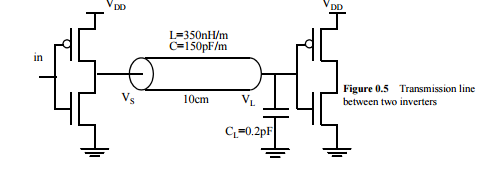# [C, SPICE, 4.x] Assume VDD=1.5V. Also, use short-channel transistor models forhand analysis. a. The...[C, SPICE, 4.x] Assume VDD=1.5V. Also, use short-channel transistor models forhand analysis.a. The Figure 0.5 shows an output driver feeding a 0.2 pF effective fan-out of CMOS gates through a transmission line. Size the two transistors of the driver to optimize the delay. Sketch waveforms of VS and VL, assuming a square wave input. Label critical voltages and times.

b. Size down the transistors by m times (m is to be treated as a parameter). Derive a first order expression for the time it takes for VL to settle down within 10% of its final voltage level.Compare the obtained result with the case where no inductance is associated with the wire.Please draw the waveforms of VL for both cases, and comment. c. Use the transistors as in part a). Suppose CL is changed to 20pF. Sketch waveforms of VS and VL, assuming a square wave input. Label critical voltages and instants.

d. Assume now that the transmission line is lossy. Perform Hspice simulation for three cases: R=100 Ω/cm; R=2.5 Ω/cm; R=0.5 Ω/cm. Get the waveforms of VS, VL and the middle point of the line. Discuss the results

• Posted: a month ago
• Due:
• Budget: \$5